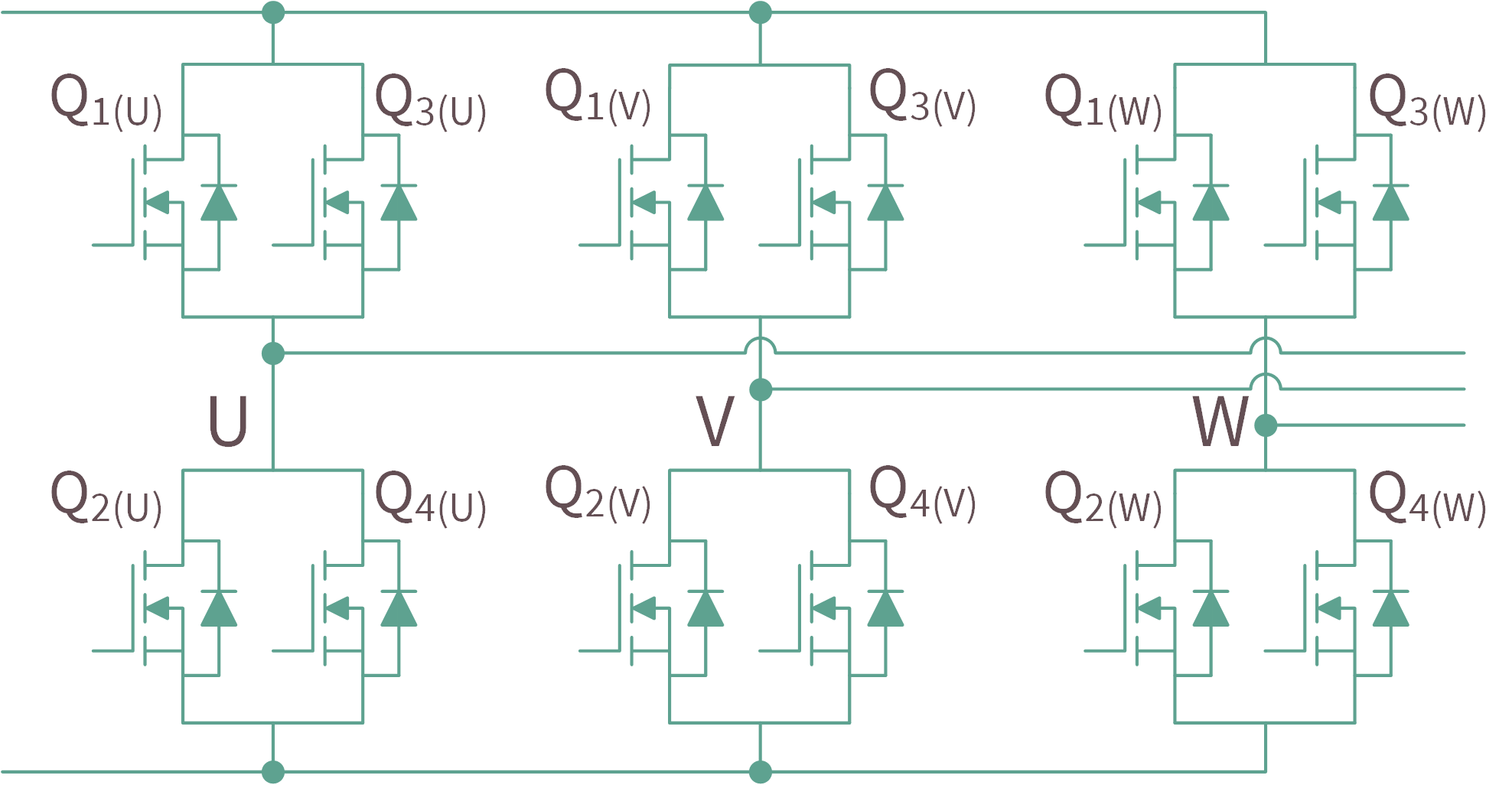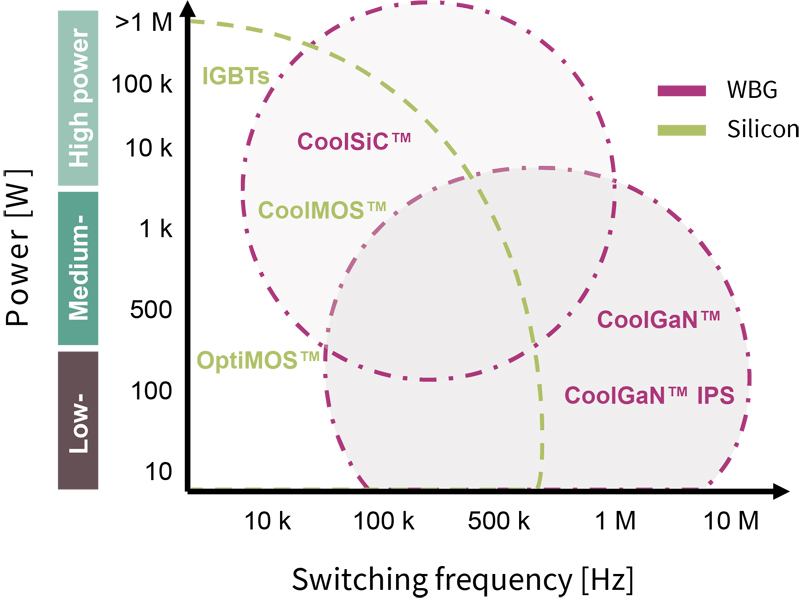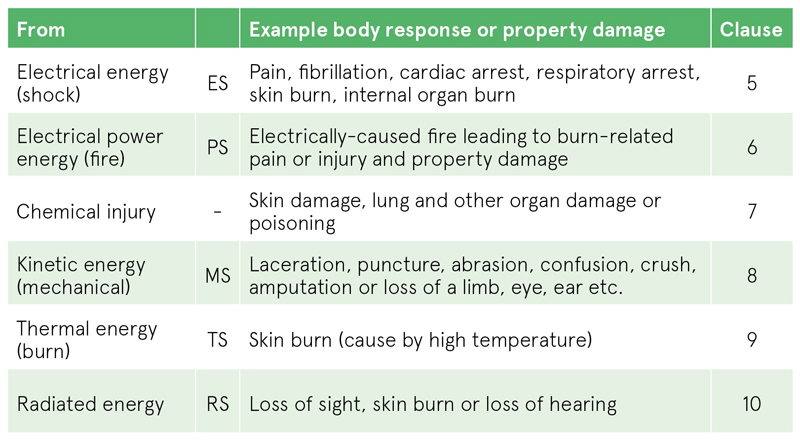# High Power Systems

Author:
Marcus O’Sullivan Applications engineer, Power Management Group, Analog Devices

Date
01/02/2014PDF
##### High accuracy Hot-Swap and power monitoring

Many high power systems require the use of a hot-swap device to safely control of the inrush currents at powerup and provide fault protection.

These circuits are commonly found in systems such as servers, network switches, redundant-array-of-independent-disk (RAID) storage, and other forms of communications infrastructure that need to remain fully operational through their working life. These systems are known as high-availability systems. In the event of a component failure, the component will need to be removed from the system and replaced with a fully functional component, all while the power remains on and system remains operational. This process is known as hot swapping, or hot plugging.

In order to safely facilitate this event, a hot-swap controller is required to control the inrush current and prevent interruption of the backplane supply to other systems. During normal operation the controller also provides protection against short circuits and other overcurrent faults. Analog Devices latest range of hot-swap controllers, also integrate a high accuracy digital power monitor which can facilitate high accuracy system power metering. See Figure 1.

Click image to enlarge

Figure 1

As the power requirements for these systems are growing, efficiency is becoming more significant. Also the practicality of designing around loose tolerance and high insertion power loss is becoming more difficult. The ADM1275 not only provides high accuracy power monitoring to report the system power, but also has many features specifically designed to reduce the losses typically associated with hot-swap, such as insertion losses of sense resistors and MOSFETs.

Let’s look at the design process, including component selection considerations, for a typical high current blade server hot-swap design.

System Specifications

The following conditions are assumed for this example:

• VIN = 12 V Nominal
• VMAX = 12.6 V
• ITRIP = ~70 A
• TAMAX = 65°C
• RPOWERUP = 10Ω (static load resistance during system power up)

To simplify this example, the calculations exclude many component tolerances. These tolerances should of course be considered when designing for worst-case conditions.

Sense Resistor Selection

The sense resistor chosen is primarily based on the required circuit breaker trip current. However, the ADM1275 also includes an adjustable current limit threshold which allows for fine tuning of the current limit beyond that provided by the limited availability of standard sense resistor values. The sense voltage can be programmed within a 5mV to 25mV range. Such a low sense voltage, along with the flexibility of programmability, offers reduced power loss and size in sense resistor selection.

The circuit breaker timer (current fault glitch filter) begins is typically 0.8mV below the regulation point. This means that to set a trip point of 70A(19.2mV) we need to set the regulation point to ~73A (20mV).

This is not a common available value so the closest to consider is 0.25mΩ, with 2x 0.5mΩ in parallel. Lets reverse the equation above to determine the required sense voltage.

The ISET pin can be programmed to a desired voltage using a divider from the VCAP reference. See Figure 2.ISET voltage = Vsense x 50.

Click image to enalrge

Figure 2

Using the VCAP reference of 2.7V and assuming R1= 100kΩ, this will result in a bottom resistor of 51.1kΩ. The given ISET voltage provides a circuit breaker trip point of ~70A and a regulation current set point of 73A.

Assuming worst case DC current could be as high as 75A (including tolerances), the maximum DC current for each resistor can be given as ~42A, including ~10% to account for imbalance.

Therefore power can be calculated as:

So each sense resistor should be capable of dissipating >1 W (including temperature derating factors). A 2W or 3W resistor is recommended to reduce running temperatures.

A series of 10Ω resistors should be used to average all these nodes together to the controller.

Summary of the key component selection for this section:

RISET(TOP) = 100 kΩ

RISET(BOT) = 51.1 kΩ

RSENSEx = 0.5mΩx2 (2 / 3W)

RAVGx = 10Ω x4

MOSFET Selection

The first consideration as criteria for selection of a suitable MOSFET is the RDSON specification, to ensure that minimum power is lost in the MOSFET when it is fully enhanced in normal operation.

The ADM1275 features a high voltage gate drive to ensure a minimum of 10V VGS is achieved to maintain the lowest specified RDSON. The gate drive circuit is designed to achieve this while still ensuring the 20V maximum VGS spec is not violated during fault conditions.

As the temperature of the MOSFET increases, its power rating is reduced, or derated. The RDSON spec determines the maximum junction temperature of the MOSFET and therefore the required derating can be applied to SOA parameters. In addition, running MOSFETs at high temperatures may decrease their reliability.

Let’s begin by estimating the required RDSON. Recall the maximum DC current was 75A, worst case. Then using the maximum ambient temperature specified in section 1 we can estimate the power loss in the MOSFET(s).

First we make a few assumptions:

·         RthJA = 40 C/W (maximum)

·         TjMAX = 120 °C

(This is the maximum preferred junction temp, keeping well away from any silicon limits)

Calculate the junction temperature rise:

Then the power for a single FET:

Now total RDSON:

This number is far too small for a single FET so let’s try 3 FETs in parallel:

Now we subtract 10% to give us some margin for imbalance due to layout asymmetry and a further 1.4 factor to allow some derate:

Taking this as our target RSDON we can now search for suitable candidates. The search can be narrowed to FETs that fit the following profile:

• VDS = 25/30V (20V may be possible but not preferred)
• VGS = 20V
• RDSON ≤ 1.4mΩ

After selecting a suitable MOSFET, the derating of the RDSON should be quantified using the MOSFET datasheet graph of RDSON against TJ

Click image to enlarge

Figure 3

Using TjMAXof 120 °C, we can see from Figure 3.the RDSON increases by a factor of ~1.52, to about 1.824mΩ(assuming 1.2mΩ at 25°C) at 120°C.  As a rule its preferred to keep junction temp ≤120C to increase reliability.

Assuming that the MOSFET’s max RDSON is 1.83mΩ, the power of each FET can be:

This is determined by the MOSFET’s thermal resistance at ambient temperature should be specified in the datasheet. The footprint size, airflow, nearby heat sources and additional copper will also have an effect on this value so care must be taken to ensure the specified conditions are met. Assume, for this design a target of:

(Note: Care should be taken to ensure layout/airflow does not exceed this figure)

As the MOSFET is expected to dissipate ~1.39W, a worst-case temperature rise of 55.6°C above ambient can be expected as follows:

The resulting junction temp of the FET can be determined as follows:

As this is below the maximum selected value of 120°C, the risk of thermal runway should be avoided. When using multiple MOSFETs in parallel, a 10Ω resistor should be use in series with the gate of each MOSFET to prevent parasitic oscillations.

Summary of the key spec / component selection for this section:

QX                     = Selected 1.2mΩ MOSFET.

RthJA                = 40 k/W

RGATE              = 10Ω (x3)

Power Derating Factor

Now that the maximum junction temperature is verified we can determine the maximum derate factor. This number will be used to derate all the SOA parameters to verify a robust solution across temperature.

To determine the maximum expected case temperature we can use:

Now the derating factor can be calculated as follows:

Summary of the key spec / component selection for this section:

DF= 2.5

Foldback

The ADM1275 utilizes a foldback technique to protect the MOSFETs in the event of overcurrent faults or short circuits. The output voltage is monitored using a divider on the FLB pin and the current limit is adjusted based on the VDS of the MOSFET.  An example of this relationship can be seen in Figure 4.

Click image to enlarge

Figure 4

When the output voltage is at zero, there is a lower limit clamp to prevent the current limit approaching zero. This clamp is fixed at 0.2V on the FLB pin (or 4mV Vsense), which equated to ~16A on this particular design. As the output voltage increases, the current limit ramps as a function of the output voltage. This threshold is set by the divider on the FLB pin, using a reference equal to VSENSEREG X 50. This voltage should be chosen to be low enough to avoid any expected VOUT load steps from affecting the current limit. The PWRGD output is also derived from the voltage level at the FLB pin.

Targeting 10.3V, we get a divider of 100KΩ top and 12KΩ bottom.

Summary of the key spec / component selection for this section:

VPG                   = 10.3V

RFLB_TOP          = 100kΩ

RFLB_BOT          = 12kΩ

MOSFET Safe Operating Area analysis

The next step is to review the SOA curve on the MOSFET datasheet to determine how much time it can tolerate the worst case power in the FET. This will determine a suitable timer capacitor value.

In a multiple FET solution, it must be assumed that a single FET could be dissipating 100% of the power during a powerup or vent such as these. This is due to possible differing Vth levels on each FETs, only one could be conducting when in regulation.

If a short was applied the Vds of the FET can be assumed to be ~12.6V (assuming source at GND). In reality the number would likely to be lower than this due to line impedance.

However, if we look at the profile of the FET power against Vout we see that the relationship is not monotonic. See Figure 5.

Click image to enlarge

Figure 5

The worst case power in the FET is shown at approximately 6.3V (50% of Vin). The current can be easily calculated using the following equation:

(WCP=Worst Case Power)

Now derate this by Derate Factor of 2.5 and we get 135A. So if we go to the SOA diagram of the MOSFET, and intersect 6.3V with 135A, we get approximately 0.8ms. See Figure 6.

Click image to enlarge

Figure 6

It should be noted that some FETs SOA power lines do not always represent a constant power product. This should be checked and if the line is not constant power then more points should be checked. For example, check VMAX of 13.2V against IFLBMIN = 16 A, derated to 40A. In this case the 6.3V SOA is very similar. If there is no specific requirement for the fault filter, its recommended to further reduce this to account for SOA tolerances and inaccuracies. In this case, lets reduce by 50% to 0.4ms.

Summary of the key spec / component selection for this section:

TSOA_MAX         = 400µs

Powerup analysis

Now that the timer had been selected, we must check to verify that there is sufficient time available to allow the loads caps to complete powerup. This is determined by how long the startup current profile intersects with the current limit… i.e. how long the timer is active during powerup.

During the power-up phase, the controller will usually hit the current limit due to the inrush current demanded by the load capacitance. If the time set by the TIMER pin is insufficient to allow the load capacitors to charge, then the MOSFET will be disabled and system will not power up. We can use the following equation to estimate the powerup time using an average current limit across the foldback system:

As the time required exceeds the determined SOA limits, the system would not complete powerup into this size load capacitance. To overcome this the inrush current needs to be lowered to a level below the hotswap control limit at powerup. This is achieved by increasing the effective gate capacitance resulting in a slower powerup time and a lower inrush current. In this way the inrush is controlled using an open loop source follower system. To avoid exceeding the current limit(16A), an additional gate capacitor can be determined as follows to provide an inrush of ~10A:

The effective CGD of the MOSFETs can be subtracted from this figure. However, to account for tolerances we can round up to 15nF.

Powerup time can now be calculated as follows:

Summary of the key spec / component selection for this section:

CGATE              = 15nF

TPOWERUP         =~ 7.5ms

Timer Capacitor

Now that the MOSFETs SOA requirements have been determined, and powerup time is satisfied, a TIMER capacitor value can be calculated. This can be calculated as follows:

Where ITIMER = 60 μA and VTIMER = 1.0 V,

Power in MOSFET at startup

Now, as a final step we need to check that the power being dissipated in the FETs at startup is within the SOA limits of the MOSFET. We can calculate the Energy required to charge the load capacitor as follows:

The power can be determined using

(where t = 7.5ms)

Derate this to 133W. Now calculate the current at average Vds (VINMAX / 2):

Now if we examine the SOA again, we can see that 6.3V and 22A corresponds to >10ms, satisfying SOA limitations.

Design Complete!

click image to enlarge

Figure 7

Here in Figure 7 is the complete design for the main circuit components.

### RELATED#### High-Current Designs Utilizing Paralleled MOSFETs in Motor Drive Applications

Sep 8,2021#### Integration is What You Need

Sep 1,2021#### Five Key Factors for Choosing Wireless IIoT Gateways for Energy Providers

Sep 1,2021Sep 1,2021